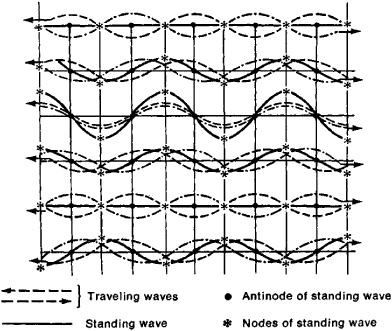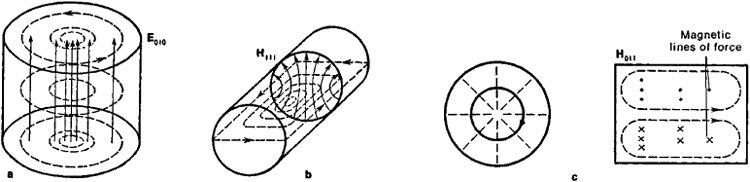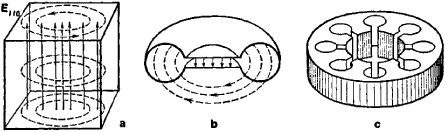# Cavity Resonator

Also found in: Dictionary, Thesaurus, Medical, Wikipedia.

## cavity resonator

[′kav·əd·ē ′rez·ən‚ād·ər]
(electromagnetism)
A space totally enclosed by a metallic conductor and excited in such a way that it becomes a source of electromagnetic oscillations. Also known as cavity; microwave cavity; microwave resonance cavity; resonant cavity; resonant chamber; resonant element; rhumbatron; tuned cavity; waveguide resonator.

## Cavity Resonator

an oscillatory system that operates at superhigh frequencies; it is the analog of an oscillatory circuit.

The cavity resonator has the form of a volume filled with a dielectric—air, in most cases. The volume is bounded by a conducting surface or by a space having differing electrical or magnetic properties. Hollow cavity resonators—cavities enclosed by metal walls—are most widely used. Generally speaking, the boundary surface of a cavity resonator can have an arbitrary shape. In practice, however, only a few very simple shapes are used because such shapes simplify the configuration of the electromagnetic field and the design and manufacture of resonators. These shapes include right circular cylinders, rectangular parallelepipeds, toroids, and spheres. It is convenient to regard some types of cavity resonators as sections of hollow or dielectric wave guides limited by two parallel planes.

The solution of the problem of the natural (or normal) modes of oscillation of the electromagnetic field in a cavity resonator reduces to the solution of Maxwell’s equations with appropriate boundary conditions. The process of storing electromagnetic energy in a cavity resonator can be clarified by the following example: if a plane wave is in some way excited between two parallel reflecting planes such that the wave propagation is perpendicular to the planes, then when the wave arrives at one of the planes, it will be totally reflected. Multiple reflection from the two planes produces waves that propagate in opposite directions and interfere with each other. If the distance between the planes is L = nλ/2, where λ is the wavelength and n is an integer, then the interference of the waves will produce a standing wave (Figure 1); the amplitude of this wave will increase rapidly if multiple reflections are present. Electromagnetic energy will be stored in the space between the planes. This effect is similar to the resonance effect in an oscillatory circuit.Figure 1. Formation of standing wave in space between two parallel planes as a result of interference between direct wave and reflected wave

Normal oscillations can exist in a cavity resonator for an infinitely long time if there are no energy losses. However, in practice, energy losses in a cavity resonator are unavoidable. The alternating magnetic field induces electric currents on the inside walls of the resonator, which heat the walls and thus cause energy losses (conduction losses). Moreover, if there are apertures in the walls of the cavity and if these apertures intersect the lines of current, then an electromagnetic field will be generated outside the cavity, which causes energy losses by radiation. In addition, there are energy losses within the dielectric and losses caused by coupling with external circuits. The ratio of energy that is stored in a cavity resonator to the total losses in the resonator taken over one oscillation is called the figure of merit, or quality factor, or Q, of the cavity resonator. The higher the figure of merit, the better the quality of the resonator.

By analogy with wave guides, the oscillations that occur in a cavity resonator are classified in groups. In this classification, the grouping depends on the presence or absence of axial and radial (transverse) components in the spatial distribution of the electromagnetic field. Oscillations of the H (or TE) type have an axial component in the magnetic field only; oscillations of the E (or TM) type have an axial component in the electric field only. Finally, oscillations of the TEM type do not have axial components in either the electric or the magnetic field. An example of a cavity resonator in which TEM oscillations can be excited is the cavity between two conducting coaxial cylinders having end boundaries that are formed by plane conducting walls perpendicular to the axis of the cylinders.

Cylindrical cavity resonators are the most widely used type of cavity resonator. The types of oscillation in cylindrical cavity resonators are characterized by the three subscripts m, n, and p that correspond to the number of half waves of the electric or magnetic field that fit along the diameter, circumference, and length of the resonator, for instance, Emnp or Hmnp. The type of oscillation (E or H) and the subscripts of the oscillation define the structure of the electric and the magnetic field in a resonator (Figure 2). The H011 mode of a cylindrical cavity resonator exhibits a peculiar property: it is quite insensitive to whether or not the cylindrical walls and the end walls are in contact. In this mode, the magnetic lines of force are directed (Figure 2, c) such that only currents along the lateral surface of the cylinder perpendicular to the axis are excited in the walls of the resonator. This fact makes it possible for nonradiating slots to be introduced into the side walls and end walls of the cavity.Figure 2. Simplest modes of oscillation in a circular cylindrical hollow resonator: (a) E010, (b) H111, and (c) H011. Solid lines denote lines of force of electric field; broken lines denote lines of force of magnetic field. The density of the lines of force is a measure of the field intensity. For the modes E010 and H111, the density of the lines is a maximum on the axis of the cylinder (an antinode) and is equal to zero on the walls of the cylinder (a node). The lines of force of the magnetic field are closed curves.

Resonators of other shapes are sometimes used in addition to cylindrical cavity resonators; for instance, rectangular cavity resonators are used in laboratory equipment (Figure 3, a). Another important design is the toroidal cavity with a capacitive gap (Figure 3, b); this resonator is used for the oscillatory system of the klystron. The fundamental mode of such a cavity resonator is distinguished by the fact that the electric field and the magnetic field are spatially separated. The electric field is localized mainly in the capacitive gap, and the magnetic field in the toroidal cavity. The field distribution in dielectric cavity resonators is similar to the field distribution in hollow metal resonators of the same shape if the difference between the dielectric constant of the resonator and that of the surrounding space is substantial. In contrast to hollow cavity resonators, the field of dielectric resonators does penetrate into the surrounding space. This field, however, is rapidly damped with increasing distance from the surface of the dielectric.Figure 3. (a) Rectangular hollow cavity resonator in which fundamental mode E110 is excited; solid lines denote lines of force of electric field and broken lines denote lines of force of magnetic field; (b) toroidal resonator of klystron; (c) resonator system of magnetron

Hollow metal cavity resonators are usually made of metals that have a high electrical conductivity, such as silver and copper and their alloys, or else the inner surface of the resonator is coated with a layer of silver or gold. Cavity resonators with an extremely high figure of merit can be obtained by using superconducting metals (seeCRYOELECTRONICS). A cavity resonator can be tuned to a given frequency by changing the volume of the cavity by moving the walls or by inserting metal plungers, plates, or other tuning elements into the cavity. Coupling to external circuits is usually carried out through apertures in the walls of the cavity with the aid of loops, probes, and other coupling components. Dielectrics with a high dielectric constant, such as rutile and strontium titanate, have low dielectric losses and are used for dielectric cavity resonators.

Cavity resonators are widely used in engineering as the oscillatory systems of generators (klystrons, magnetrons), as filters, as frequency standards, as measuring circuits, and in various devices designed for investigating solid, liquid, and gaseous substances. They can be used in the frequency range from 109 to 10n hertz. At higher frequencies, the wavelength of the oscillations excited in a cavity resonator becomes comparable to the dimensions of the unavoidable surface defects on the walls of the cavity resonator. This fact causes a dissipation of the electromagnetic energy, a drawback that is eliminated in open resonators consisting of a system of mirrors.

### REFERENCES

De Broglie, L. V. Elektromagnitnye volny v volnovodakh i polykh rezonatorakh. Moscow, 1948. (Translated from French.)
Vainshtein, L. A. Elektromagnitnye volny. Moscow, 1957.

I. V. IVANOV and V. I. ZUBKOV

References in periodicals archive ?
Analytical formulas valid in the case of the circular metallic cavity resonator have been adopted in which a suitable empirical equivalent radius has been used.
Yom, "A Ka-band multilayer LTCC 4-pole bandpass filter using dual-mode cavity resonators," 2008 IEEE MTT-S International Microwave Symposium Digest, 1235-1238, Jun.
Here, [Omega] is the angular eigenfrequency when the sample is inserted in the cavity resonator, [[Omega].
110] SIW cavity resonator with 50-[ohm] CPW as its input/output.
In addition to the characteristic of active loss compensation, the most remarkable feature of the present scheme is that the negative values of the permittivity and permeability of such an isotropic and homogeneous left-handed medium (with atomic-scale microscopic structure units) can be tuned by adjusting an incoherent pump field, and such a scenario for achieving a tunable negative refractive index would therefore have some potentially important applications in the development of new technologies in quantum optics and photonics, including new devices such as tunable subwavelength cavity resonator and superlenses for perfect imaging and subwavelength focusing [39-41].
Module one is the sapphire loaded cavity resonator.
At lower frequencies, cavity resonators become impractical due to their excessively large dimensions.
Forced oscillations in cavity resonators," Journal of Applied Physics, Vol.
A solution to this dilemma is to realize the oscillator and a cavity resonator simultaneously in LTCC, a multilayer ceramic medium.
Sanson, "High Q Tunable Sapphire Loaded Cavity Resonator for Cryogenic Operation," Cryogenics, Vol.
The topics include fundamentals of wave propagation, cavity resonators, microwave ferrite devices, scattering parameters, antennas, microwave measurements, microwave tubes, diodes, transistors, planar transmission lines, and microwave integrated circuits.

Site: Follow: Share:
Open / Close